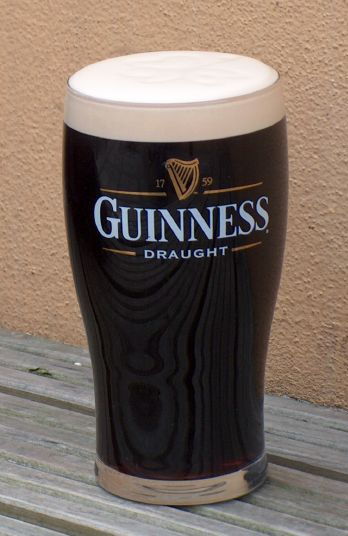Guinness Beer (By Sami Keinänen (www.flickr.com) [CC-BY-SA-2.0 (http://creativecommons.org/licenses/by-sa/2.0)%5D, via Wikimedia Commons)

The title of Chapter 25 of Zar is Testing for Randomness. Which sounds pretty philosophical.

Definition of random distribution can be applied to objects (each portion of space has the same probability of containing an object, and the occurrence of one does not influence the occurrence of the other), events in time (substitute space for time, and object for event), or periods of time.

The Poisson distribution is important in describing random occurrences when their probabilities are small.

A practical application of this distribution was made by Ladislaus Bortkiewicz in 1898 when he was given the task of investigating the number of soldiers in the Prussian army killed accidentally by horse kick; this experiment introduced the Poisson distribution to the field of reliability engineering.

(Copying here shamelessly from wikipedia): The Poisson distribution arises in connection with Poisson processes. It applies to various phenomena of discrete properties (that is, those that may happen 0, 1, 2, 3, … times during a given period of time or in a given area) whenever the probability of the phenomenon happening is constant in time or space. Examples of events that may be modelled as a Poisson distribution include, among others, the number of yeast cells used when brewing Guinness beer. This example was made famous by William Sealy Gosset (1876–1937).

This interesting fact made me digress from Poisson long enough to learn about Gosset, who seems to be a very interesting character. From Tales of  Statisticians:

Gosset earned a degree in chemistry at Oxford, and joined the Guinness brewery firm in 1899. His work for Guinness led him investigate the statistical validity of results obtained from small samples (previous statistical theory had concentrated instead on large samples). He took a leave of absence to spend 1906/1907 studying under Karl Pearson at University College, London. His publications in Pearson’s journal Biometrika were signed “Student,” not because of a Guinness company policy forbidding publication, as is often said, but more precisely because of a company wish to keep secret the fact that they were gaining an industrial advantage from employing statisticians. Gosset’s most important result is known as the “Student’s t” test or distribution, published in 1908.

Returning to Poisson_ still working on it, but found some pointers.

You will have a variable “x” which is what your random variable is,

then u is the average success (divide n by X)

and you have to use the constant e in your calculations

Poisson Formula. Suppose we conduct a Poisson experiment, in which the average number of successes within a given region is μ. Then, the Poisson probability is:

P(x; μ) = (e) (μx) / x!

where x is the actual number of successes that result from the experiment, and eis approximately equal to 2.71828.

Life became much simpler when I found an online calculator.

To be continued…